# Team:SCUT/Model/Rubisco simulation

Introduction

The “bottom up” method is a helpful tool to solve our problem. By applying it, we make the original problem simple, that is, dividing the original network into three parts, and then merging them together to build a complete system. With the guide of this principle, we choose the ODEs to model our individual part. The reason why we use the ODEs consists of two parts. One is that they are feasible to combine together to construct a whole network. The ODEs are just like components, which have interfaces to communicate with others easily. Another one is that we can simply sweep the parameters to simulate the changed experiment conditions.

The steps for our work are as follows:

1. Since the pathway consists of three parts, which are PPP(pentose phosphate pathway), glycolysis and part of Calvin Cycle, we firstly find the parameters and model them individually.

2. After making sure the correctness of our individual parts of modeling, we combine them together to figure out the performance of the pathway.

3. By comparing the difference of the production of ethanol between with and without the part of Calvin Cycle, we present the function of RuBisCo.

4. By using parameter sweep, we find out the optimal reaction rate ratio of the reactions involved in the scaffold, thus proving the function of scaffold and showing the internal limitation of the pathway.

Individual part

The ODEs is mainly based on Michaelis-Menten equation. However, the equation we used is an improved version of Michaelis-Menten equation, that is, by introducing more parameters to indicate the factors influencing the reaction. Next, let us show them individually.

1. PPP(pentose phosphate pathway)

According to what we have investigated , we build the PPP subsystem. The patterns of the equations are similar. In order to make our presentation brief, we only introduce one of the equations and recommend you to visit the BioModel Database  for detail. (For more information, please see the “Tips for other teams” part). Take GND reaction as an example, the equation of GND reaction rate and the parameters are showed as follows: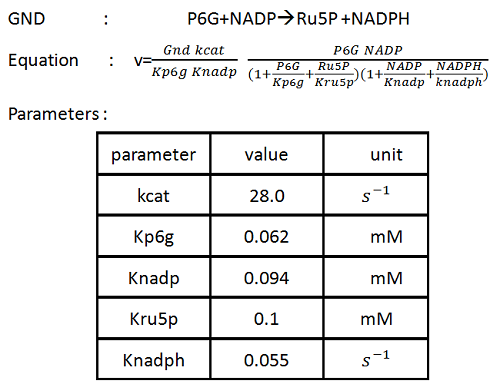The result are showed in figure 1: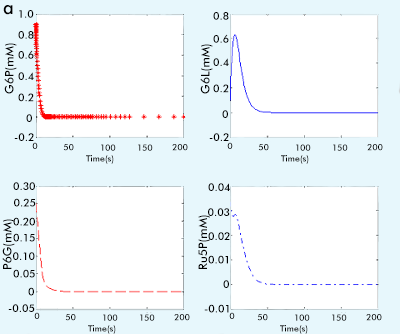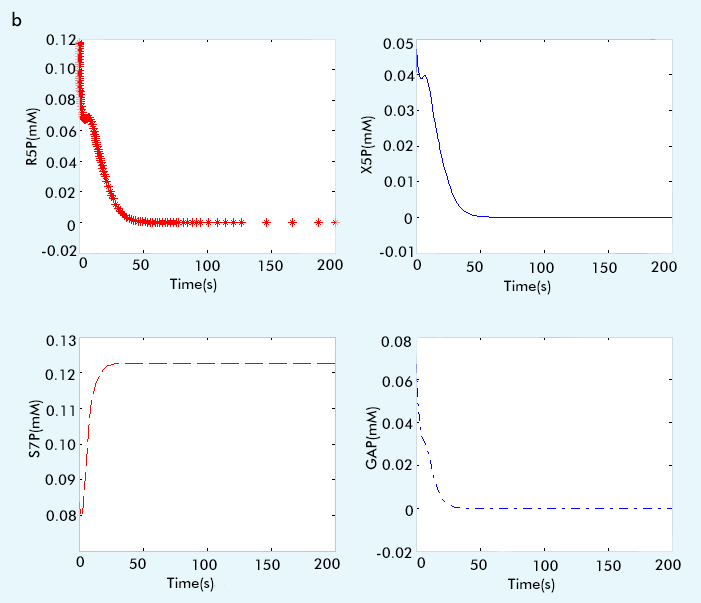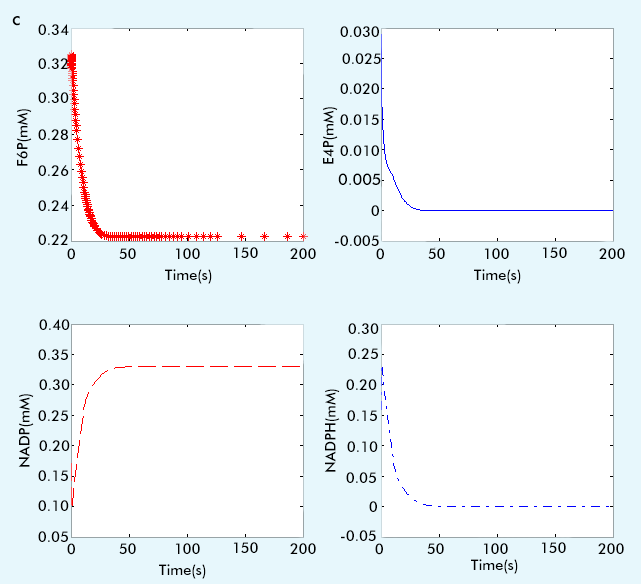Figure 1 The result of PPP subsystem

2. Glycolysis

The glycolysis in yeast is the most complex model since it consists of numerous reactions and different kinds of mechanisms. Just as what we have done in the PPP part, that is, to make the presentation brief, we will show the representation of each kind of mechanism and suggest you to browse the BioModel Database for detail.

For the reactions PGI, PGM and ENO, they can be modeled by using one substrate, one product reversible Michaelis-Menten kinetics: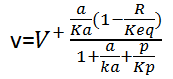where a and p represent the concentrations of the corresponding substrate and product respectively, R is the mass-action ratio, p/a, Keq is the equilibrium constant, peq/aeq. Ka and Kp are the Michaelis-Menten constants for a and p.
For the reactions HK, GraPDH, PGK and PYK, we use reversible Michaelis-Menten kinetics for two noncompeting substrate-product couples to model them: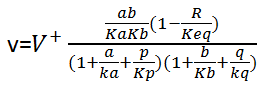where a and b represent the concentrations of the substrates , p and q represent the concentrations of the products. For the transport of glucose: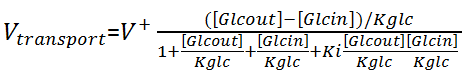where Ki is the interactive constant, [Glcout] and [Glcin] are the concentrations of extracellular and intracellular glucose respectively. For ALD, which follows an ordered uni-bi mechanism, the equation is: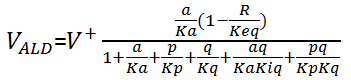where a represent the substrate [F16bP], p and q represent the products [GPP] and [GraP] respectively.
For PDC, irreversible hill kinetics are used to describe the reaction rate: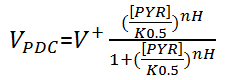For ADH, it follows ordered bi-bi kinetics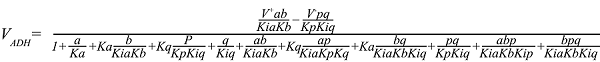For ATPase, it is the simplest one: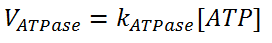For PFK, it is the most complex one: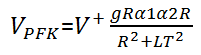with: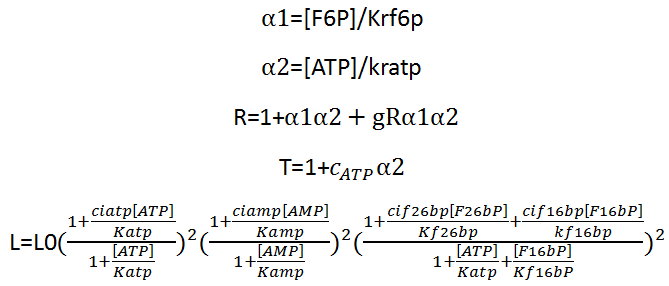After finding out the equations and parameters, we run the program and get the following result( figure 2).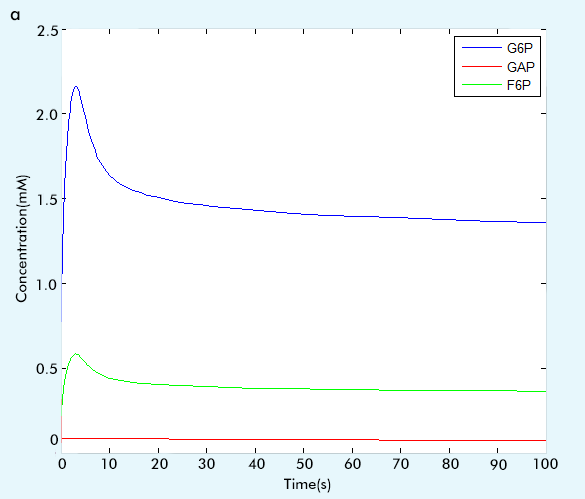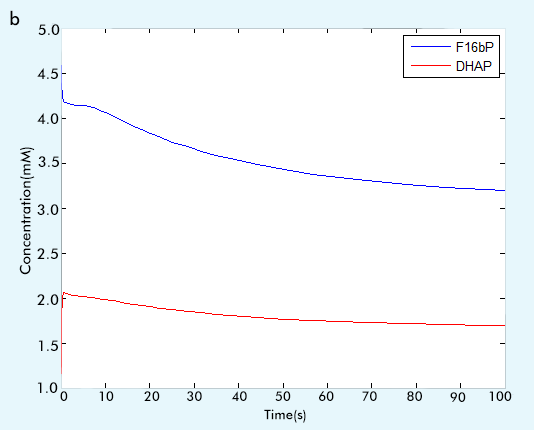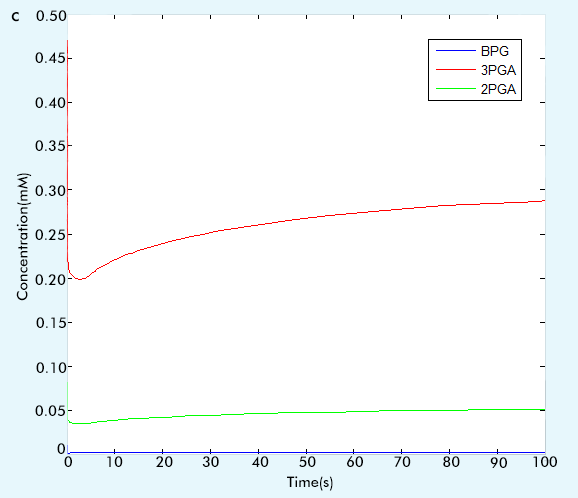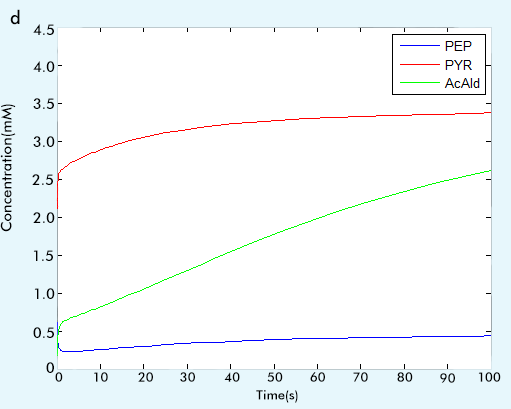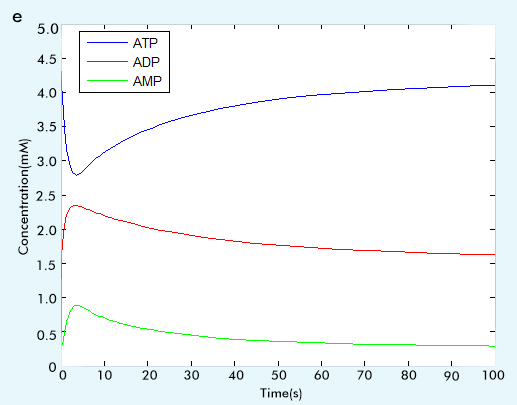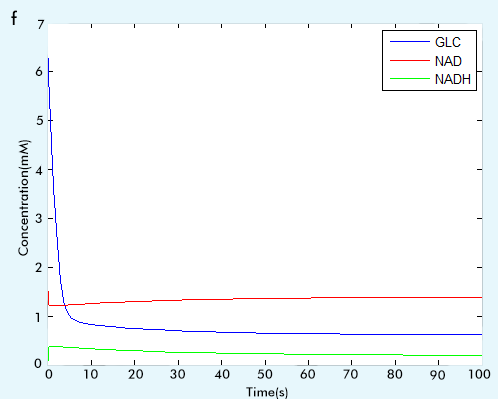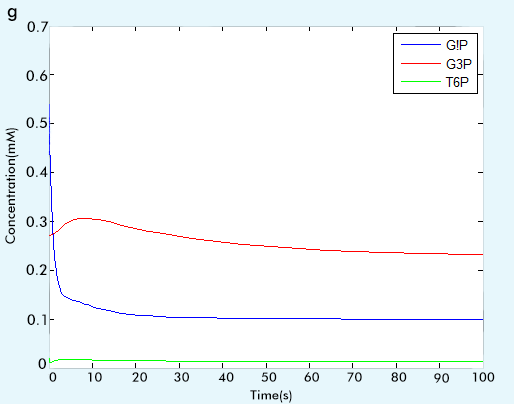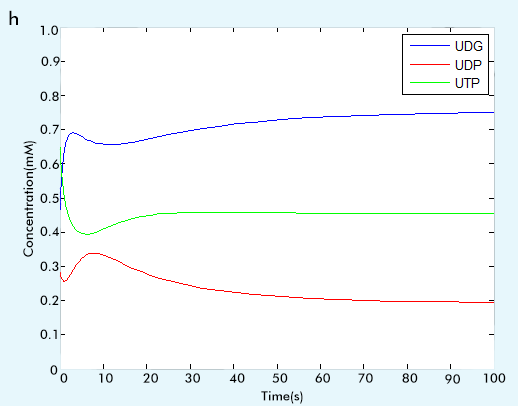Figure 2 The result of glycolysis subsystem

3. Part of Calvin Cycle

This part is the most significant part of our network since it is the part we introduce into the yeast to improve the production of ethanol. It involves two reactions, whose kinetic equations follow the classic Michaelis-Menten kinetics .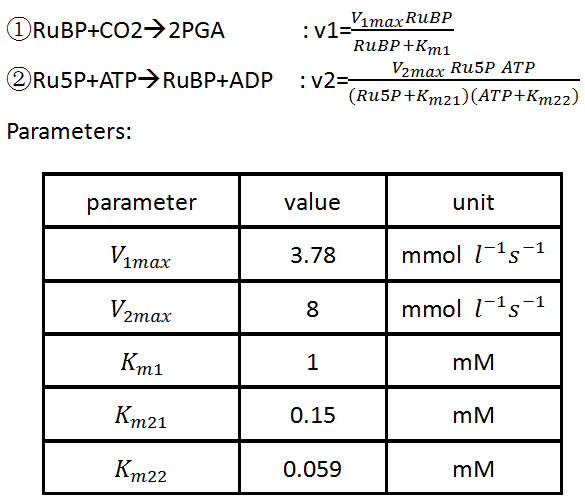This part is so small that we didn’t run it individually and we will see its function in the Complete network and The function of RuBisCo.

Complete network

After we make sure the correctness of each part, that is, by comparing the running results with the paper results or the online math lab running results  to see if our results are consistent with them.(For more information, see the part "Tips for other teams"), we combine three parts together to construct our complete network. The results of our complete network are showed in figure 3.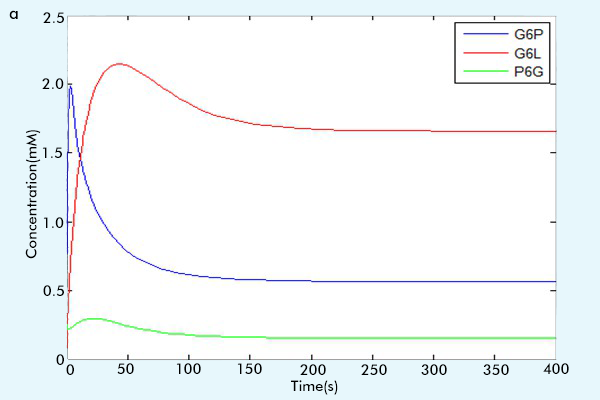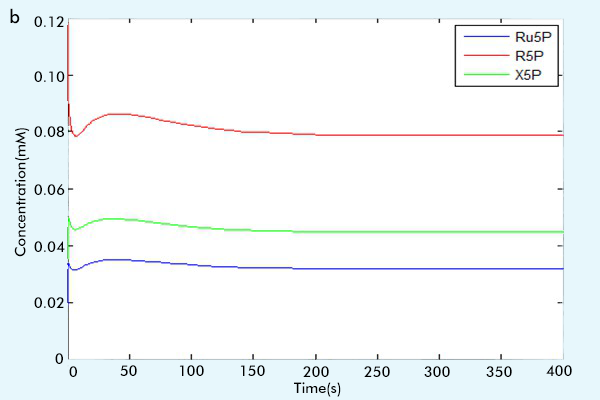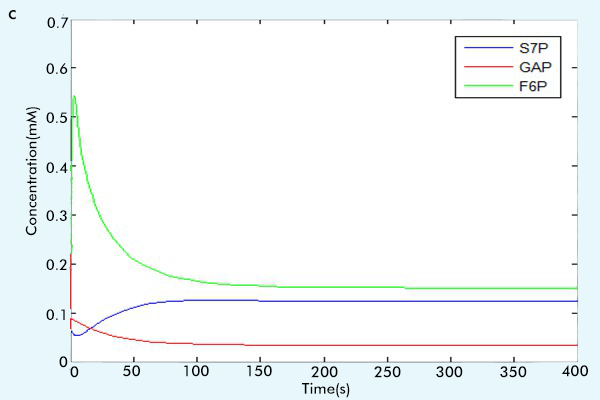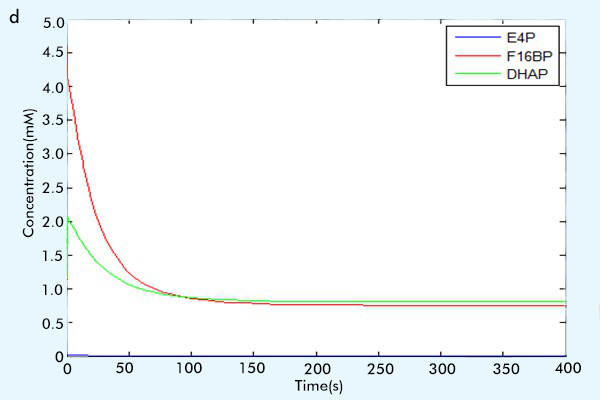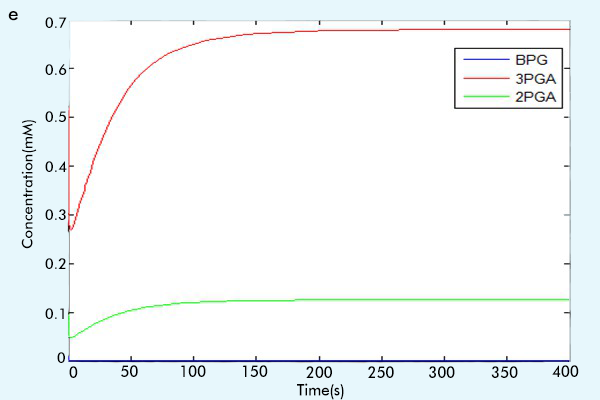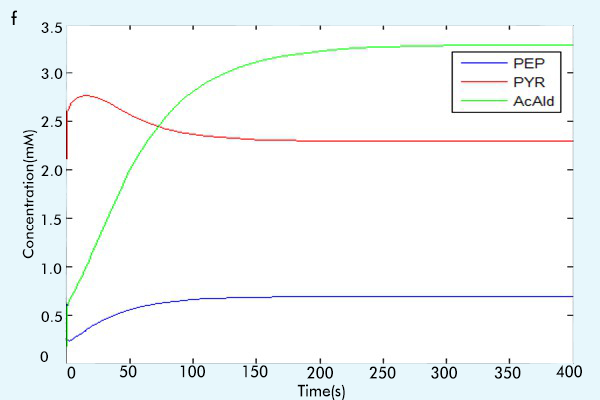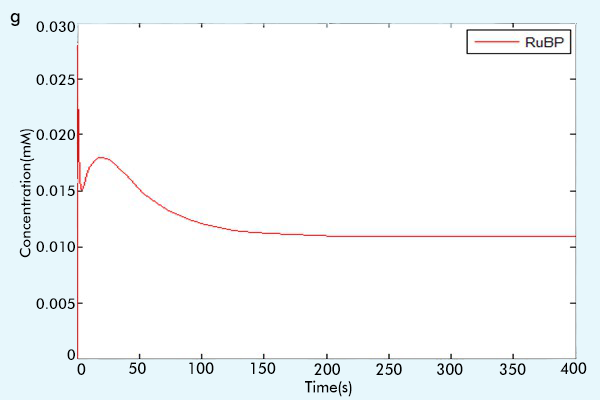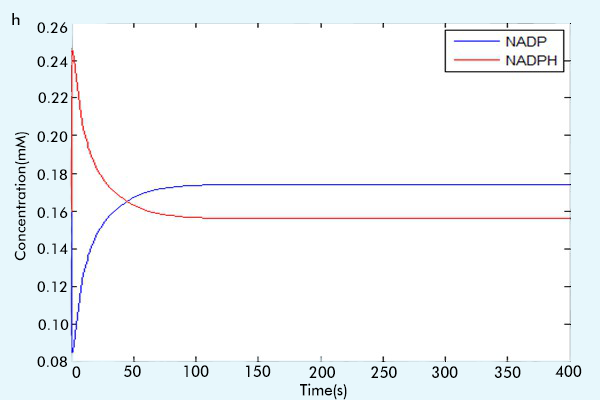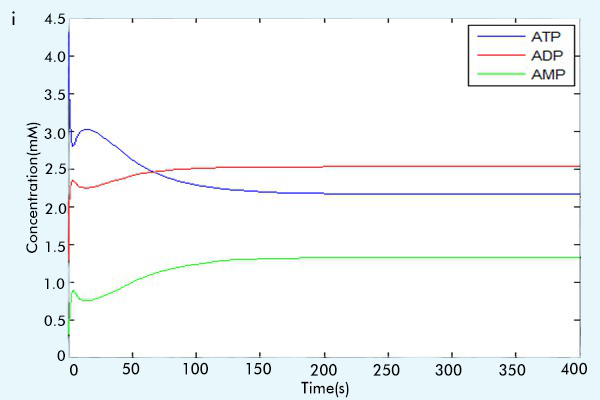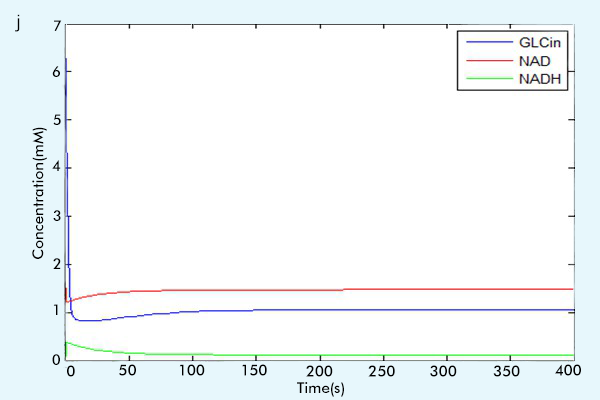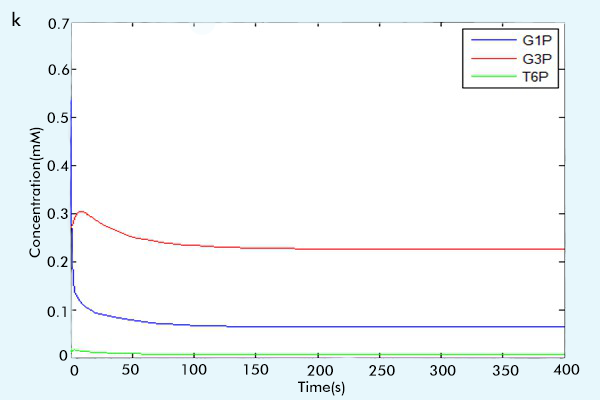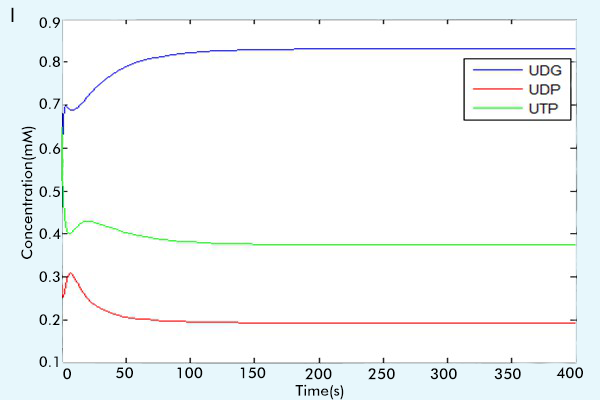Figure 3 The results of the whole system

The function of RuBisCo

In order to test the function of RuBisCo, that is, the enzyme we have introduced into the yeast, we compare the production of ethanol of two different networks, one is with the RuBisCo while the other is the original one. In fact, the network with RuBisCo is the network we have presented in the Complete work, and the original one is the network without part of Calvin Cycle. We construct two different ODEs system to see the change of production of ethanol. The comparison result shows the function of RuBisCo, which is improving the production of ethanol. From figure 4, we can see that with the introduction of RuBisCo, the ethanol yield has been increased by 8.3868%. (This data is calculated in MATLAB and displayed on the command window)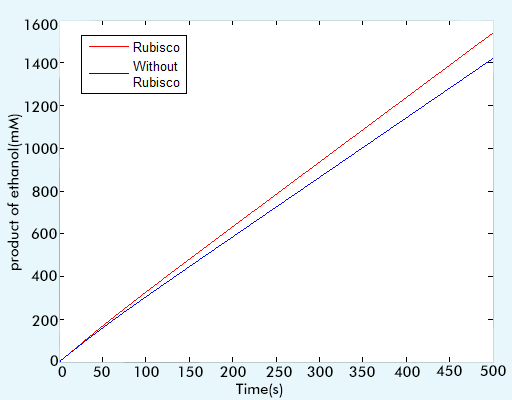Figure 4 the comparison result of the networks with and without RuBisCo

Scaffold

In addition to introduce RuBisCo into yeast, we also assemble that part on the scaffold for it can increase the effective concentrations of metabolic intermediates . As a result, we wonder the optimal reaction rate ratio, that is, the best multiples of the original reaction rate, for our network.
To find out the optimal ratio, we sweep the parameters of the reactions that assembling on the scaffold to see the change of production of ethanol. For all of the parameters to be discussed, vmax is the most important one. Consequently, we choose the vmax to sweep. We plot the production-reaction rate ratio figure to make the change more straightforward. According to the result (figure 5), we can make the conclusion that with the improvement of reaction rate ration, the product become more and more large. However, when the ratio has become 20 multiples of the original one, which is with RuBisCo but without scaffold, the change of production become small and can be ignored. So the optimal reaction rate ratio is approximately 20 multiples. And at that situation, the production of ethanol has been increased by about 5% when comparing to the one with RuBisCo but without scaffold.
Maybe you will find the trend is strange and ask, why it isn’t showed like that with the increase of reaction rate, the product also increase without limitation. To explain such strange thing, we should firstly show the reason why RuBisCo can increase the production of ethanol. It is because the RuBisCo pathway is a competitive pathway with the fermentation of glycerol . The glycerol is one of the by-products of the production of ethanol, and with the introduction of RuBisCo, we can redistribute the nutrients to the direction we want, which is improving the production of ethanol. And by using scaffold, we can enhance the performance of RuBisCo. However, the fermentation of glycerol is limited, when we achieve the better situation, that is, making the production of glycerol become zero, we cannot enhance the performance of RuBisCo anymore.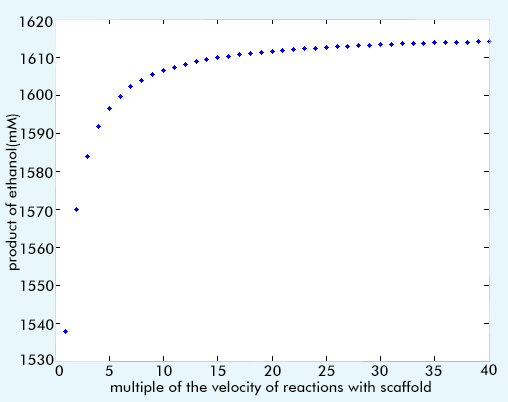Figure 5 The relationship of the product and the reaction rate ratio

Reference

 Hanan et al.: Enzyme characterisation and kinetic modelling of the pentose phosphate pathway in yeast, PeerJ PrePrints , https://peerj.com/preprints/146v1/, v1 received: 9 Dec 2013, published: 9 Dec 2013, doi: 10.7287/peerj.preprints.146v1
http://www.ebi.ac.uk/biomodels-main/, BioModels Database: An enhanced, curated and annotated resource for published quantitative kinetic models
Smallbone et al.: A model of yeast glyolysis based on a consistent kinetic characterisation of all its enzymes, FEBS Letters 587 (2013) 2832–2841
 Teusink, B. et al.: Can yeast glycolysis be understood in terms of in vitro kinetics of the constituent enzymes? Testing biochemistry, Eur. J. Biochem.267, 5313–5329
 Xin-Guang Zhu et al.: A simple model of the Calvin cycle has only one physiologic feasible steady state under the same external conditions, Nonlinear Analysis: Real World Applications 10 (2009) 1490–1499
 http://jjj.mib.ac.uk/database/smallbone, JWS online-smallbone model
 John E Dueber et al.: Synthetic protein scaffolds provide modular control over metabolic flux, NATURE BIOTECHNOLOGY, VOLUME 27 ,NUMBER 8, 753-759
 Guadalupe-Medina et al.: Carbon dioxide fixation by Calvin-Cycle enzymes improves ethanol yield in yeast, Biotechnology for Biofuels 2013 6:125